NOIP2007 普及组Actinoi 7月 22, 2019

# T1:奖学金

## 输入输出样例

6
90 67 80
87 66 91
78 89 91
88 99 77
67 89 64
78 89 98


6 265
4 264
3 258
2 244
1 237


8
80 89 89
88 98 78
90 67 80
87 66 91
78 89 91
88 99 77
67 89 64
78 89 98


8 265
2 264
6 264
1 258
5 258


NOIP2007普及组第一题

# 题解：

​ ​一道模拟题。首先，用 读入学生的语文、数学、英语三科成绩，然后用 计算总成绩，用 存储学生的编号。然后将总分从高到低进行排序，如果学号靠后的同学总成绩比学号靠前的同学的总成绩要高的话，那么交换两个学生的位置。同理，如果总成绩一样的话，再按语文成绩从高到低排序，最后，如果语文成绩也一样的话，那么学号小的同学排就在前面。

#include <cstring>
#include <iostream>
using namespace std;
int student;
void change(int i, int j) { //交换两个数组
swap(student[i], student[j]);
swap(student[i], student[j]);
swap(student[i], student[j]);
swap(student[i], student[j]);
swap(student[i], student[j]);
return;
}
int main() {
int n;
cin >> n;
for (int i = 1; i <= n; i++) {
cin >> student[i] >> student[i] >> student[i]; //语文、数学、英语成绩
student[i] = student[i] + student[i] + student[i]; //总成绩
student[i] = i; //存储学生编号
}
for (int i = 1; i <= n; i++)
for (int j = i; j <= n; j++) {
if (student[i] < student[j]) //按总分从高到低排序
change(i, j);
else if (student[i] == student[j]) {//总分相同
if (student[i] < student[j]) //按语文成绩从高到低排序
change(i, j);
else if (student[i] == student[j] && student[i] > student[j])  //学号小的同学排在前面
change(i, j);
}
}
for (int i = 1; i <= 5; i++)
cout << student[i] << " " << student[i] << endl;
return 0;
}


# T2:纪念品分组

## 输入输出样例

100
9
90
20
20
30
50
60
70
80
90


6


NOIP2007普及组第二题

# 题解：

​ ​本题是一道典型的贪心问题。我们在读入物品的价格之后，对物品的价格进行排序。然后从价格最小的物品开始枚举，从价值最大的物品反向查看能否与这个物品分为一组。为什么要从价格最大的物品开始进行查看，而不是从价格最小的物品开始呢？我们可以举一个🌰。如：

​ ​

​ ​我们看这个数组，当 时，在满足每组最多只能包括两件纪念品的要求下，如果从最小的物品开始配对，那么就需要分 组，这明显不是最优的。但是，从价格最大的物品开始配对时，仅需要 组就能满足要求。这便是最优的方案。

​ ​最后，我们再扫一遍这个数组，如果有物品没有被分组，那么就只能让这个物品单独一组了。所以说答案 就等于已经分的组数 没有被分组的物品的数量。

#include <iostream>
#include <algorithm>
using namespace std;
int things;
bool vis; //存储这个纪念品是不是已经被选了
int main() {
int w, n; //w表每组纪念品价格之和的上上限，n表购来的纪念品的总件数
cin >> w >> n;
for (int i = 1; i <= n; i++)
cin >> things[i];
sort(things + 1, things + 1 + n); //将纪念品价格从高到低排序
int ans = 0; //一共需要分几组
for (int i = 1; i <= n; i++)
if (vis[i] != true)
for (int j = n; j > i; j--) { //在不超过w元的情况下，尽可能让每组的钱数最大
if (vis[j] != true && things[i] + things[j] <= w) {
ans++;
vis[i] = true;
vis[j] = true;
break;
}
}
for (int i = 1; i <= n; i++)
if (vis[i] != true) //有一些纪念品无法两个一组，就单独一组
ans++;
cout << ans << endl;
return 0;
}


# T3:守望者的逃离

## 输入输出样例

39 200 4


No
197


36 255 10


Yes
6


NOIP2007普及组第三题

# 题解：

​ ​对于本题，我们读过题目之后可以想到，这是一个动态规划问题。守望者在逃离海岛时，要么使用闪烁法术，要么跑步。因此，我们便可以考虑分别 这两种情况。

​ ​单独考虑第一种情况，我们可以想到，如果守望者的魔法值 的话，那么便使用闪烁法术，使 。而如果无法使用闪烁法术的话，那么便便令 ，然后

​ ​然后我们再考虑第二种情况。由于 数组目前已经有值，所以，对于第二种情况的 数组而言，要么继续保持现在的数值，要么将上一秒所能够跑的最大路程 ,我们取一个 值，便得到了。最后，统计一下答案就好辣！

#include <iostream>
using namespace std;
int DP;
int main() {
int m, s, t; //魔法初值m，他所在的初始位置与岛的出口之间的距离s，岛沉没的时间t
cin >> m >> s >> t;
for (int i = 1; i <= t; i++) {
if (m >= 10) {
m -= 10;
DP[i] = DP[i - 1] + 60;
} else {
//如果不够减
DP[i] = DP[i - 1];
m += 4;
}
}
int maxx = 0; //守望者能走的最远距离
for (int i = 1; i <= t; i++)
DP[i] = max(DP[i], DP[i - 1] + 17);
for (int i = 1; i <= t; i++) {
if (DP[i] >= s) {
cout << "Yes" << endl;
cout << i << endl;
return 0;
}
if (DP[i] > maxx)
maxx = DP[i];
}
cout << "No" << endl;
cout << maxx << endl;
return 0;
}


# T4:Hanoi 双塔问题

## 题目描述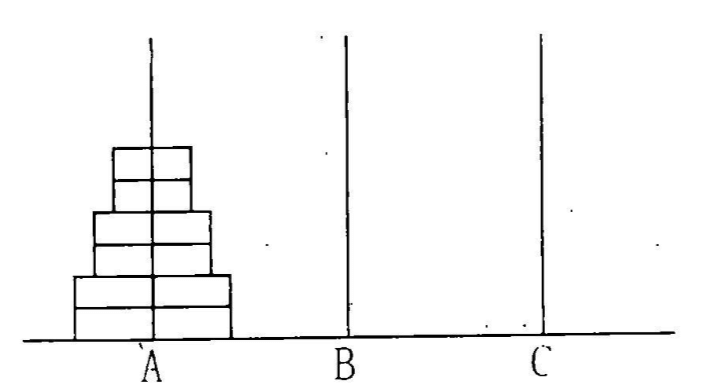（1）每次只能移动一个圆盘；

（2）三根细柱上的圆盘都要保持上小下大的顺序；

## 输入输出样例

【输入样例1】
1
【输入样例2】
2


【输出样例1】
2
【输出样例2】
6


【限制】

【提示】

NOIP2007普及组第四题

# 题解：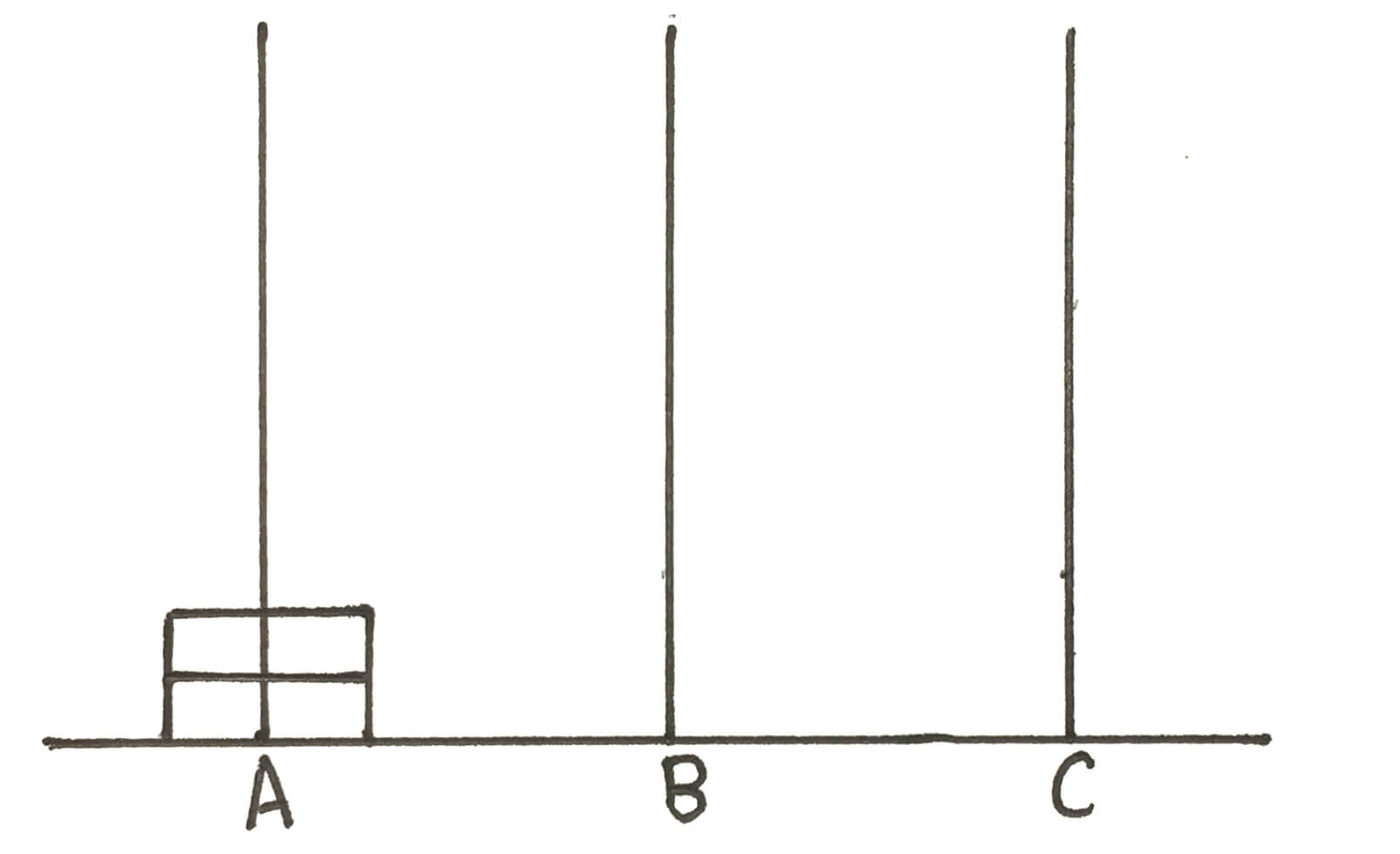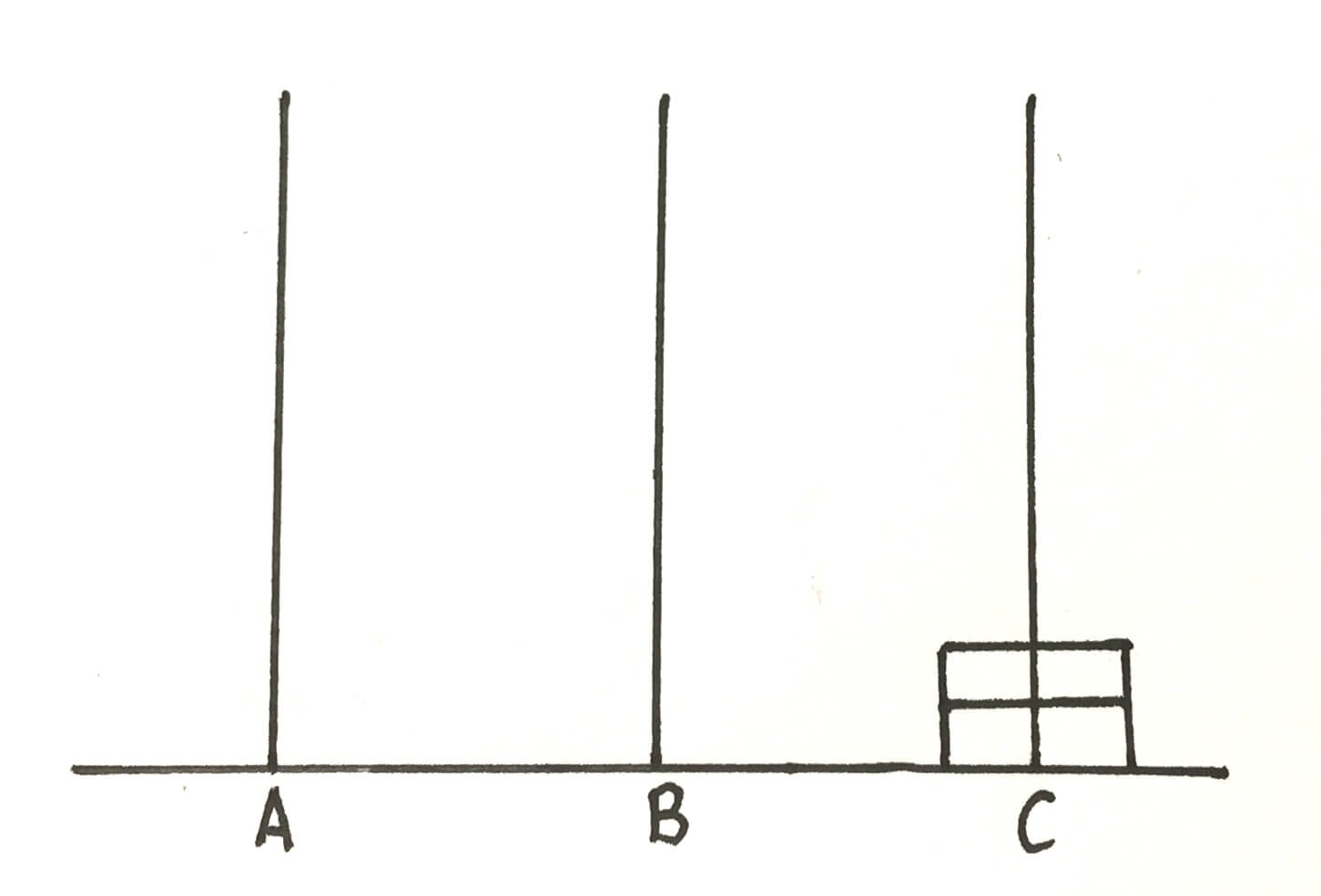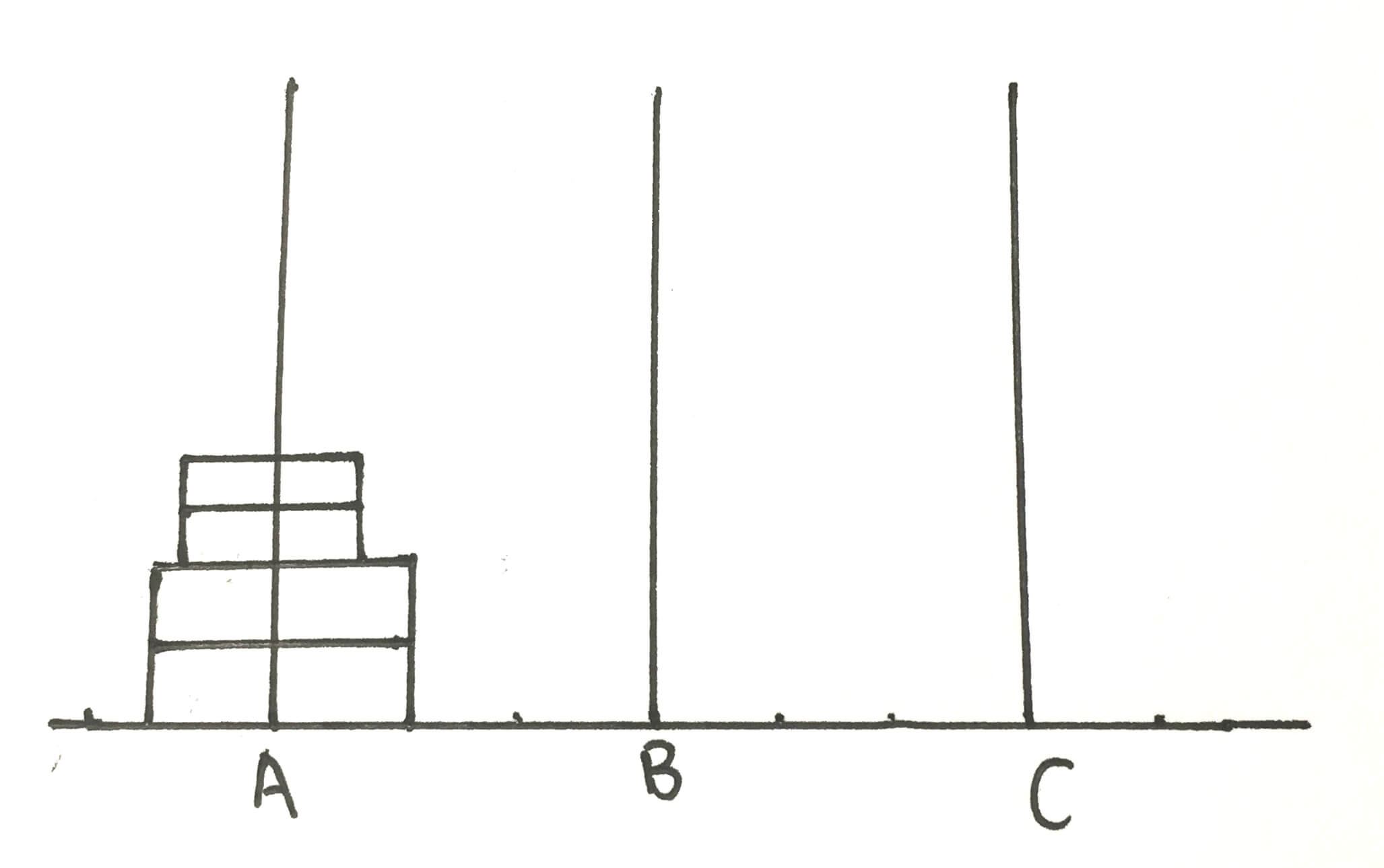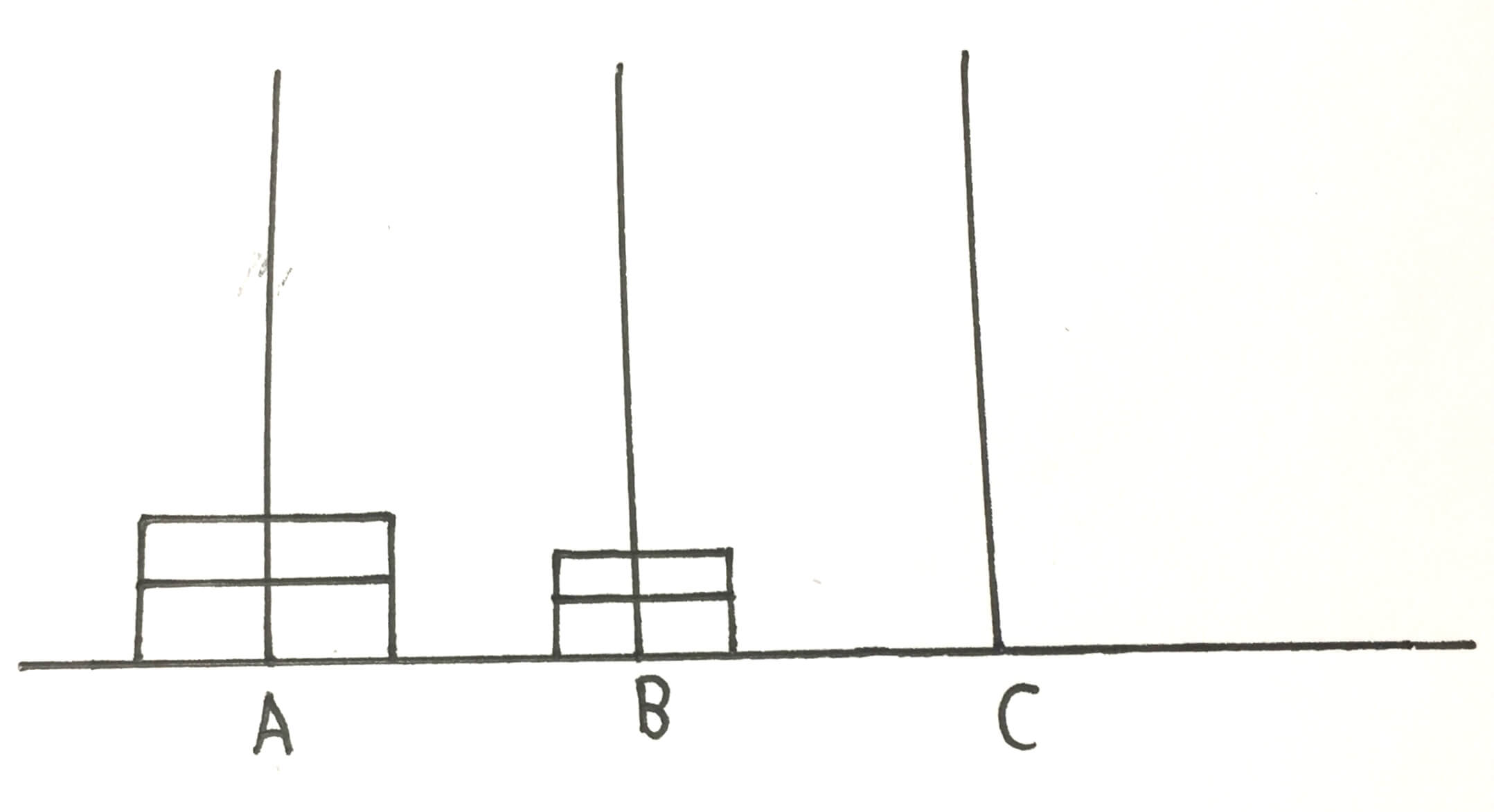（是不是这个过程与 的移动过程完全一样？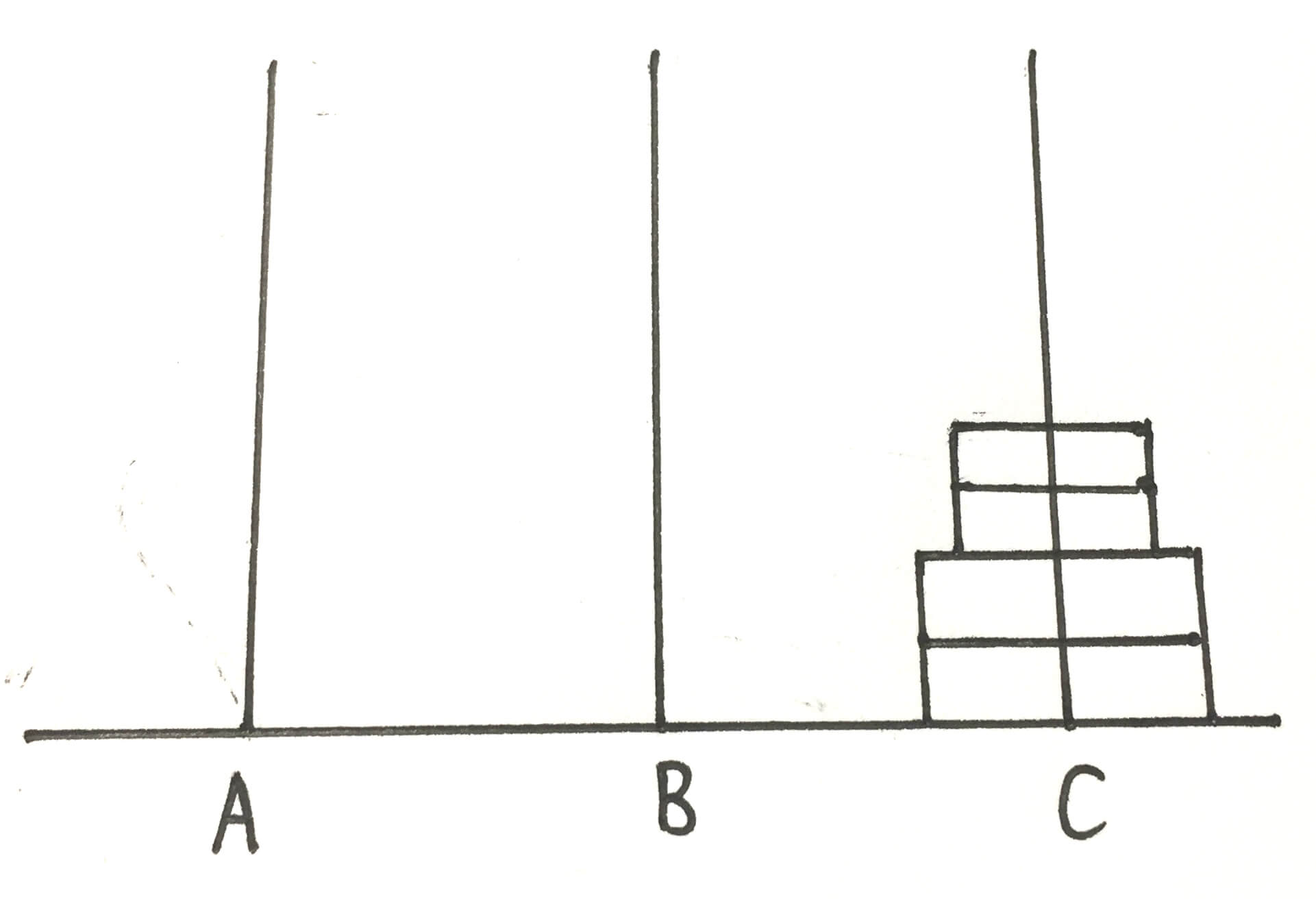n 1 2 3 4 5

#include <iostream>
using namespace std;
int Hanoi1, Hanoi2; //滚动数组
int w = 1; //记录位数
void move(int i) {
if (i % 2 == 1) {
for (int i = 1; i <= w; i++) //高精度乘法
Hanoi1[i] = Hanoi2[i] * 2;
Hanoi1 += 2;
for (int i = 1; i <= w; i++) //高精度进位
if (Hanoi1[i] >= 10) {
Hanoi1[i + 1] += Hanoi1[i] / 10;
Hanoi1[i] = Hanoi1[i] % 10;
w++;
}
} else {
for (int i = 1; i <= w; i++) //高精度乘法
Hanoi2[i] = Hanoi1[i] * 2;
Hanoi2 += 2;
for (int i = 1; i <= w; i++) //高精度进位
if (Hanoi2[i] >= 10) {
Hanoi2[i + 1] += Hanoi2[i] / 10;
Hanoi2[i] = Hanoi2[i] % 10;
w++;
}
}
}
int main() {
int n;
cin >> n;
Hanoi1 = 2;
for (int i = 2; i <= n; i++) //上一次移动的数量 * 2 + 2
move(i);
bool tag = false; //处理前导0
for (int i = w; i >= 1; i--) {
if (n % 2 == 1) {
if(Hanoi1[i])
tag = true;
if (tag)
cout << Hanoi1[i];
} else {
if(Hanoi2[i])
tag = true;
if (tag)
cout << Hanoi2[i];
}
}
return 0;
}